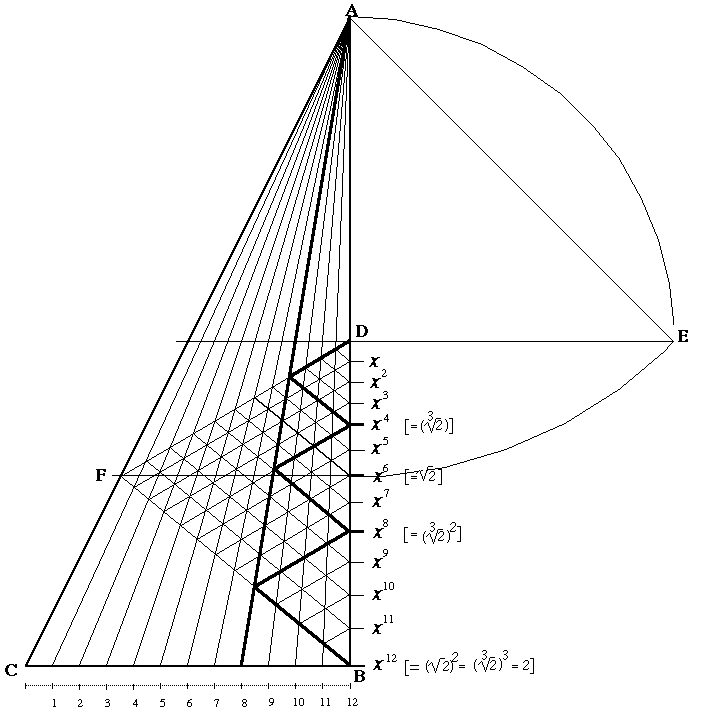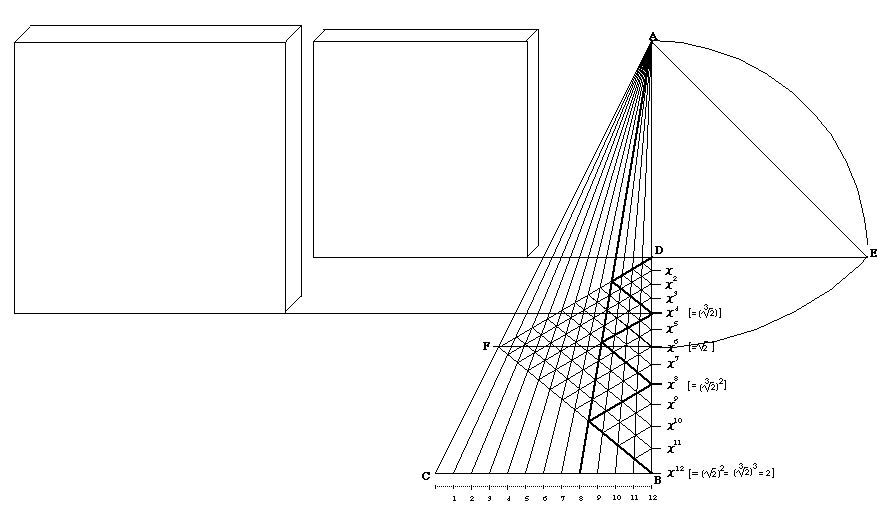Subject: Delian Problem
Date: Tue, 6 Nov 2001 15:02:53 +0000
From: Roger Wibberley

# Duplication of the Cube

### (straight edge and compass only)

Despite algebraic proofs to the contrary, this message seriously proposes a Euclidean solution to the problem of doubling the cube. It arises from a project designed to provide a Euclidean solution to the drafting of Equal Temperament, in which the octave is geometrically divided into 12 equal semitones (which is strictly contrary to the natural laws of acoustics, but which nonetheless was proposed by Aristoxenus and his followers through antiquity, and has become normal since the 19th century). No strictly geometric solution has so far been achieved. The barrier to finding a geometric solution to Equal Temperament has generally been thought to have been the inability to draft the cube root of 2 - without which the tempered major thirds were thought to be unattainable.

Having discovered a means of achieving a 12-semitone, equally-tempered scale, I am now proposing that this discovery demonstrates that the drafting of the cube root of 2 is not a prerequisite for the tempered scale, but rather arises from its solution.### Solution

Draw a right-angled ΔABC whose height (AB) is twice its width (BC), with hypotenuse AC. AB thus equals 2, and BC equals 1, and AC the square root of 5.

Bisect AB at point D so that AD = BD = BC.

At point D, errect DE perpendicular to AB such that DE = AD.

Draw a straight line from A to E.

Since AD = 1 and DE = 1, AE must equal the square root of 2. Place the compass point on A, and open it to the length AE.

With E as a point on the circumference of a circle with the radius AE, draw an arc which intersects the line AB. The point x6 where the arc intersects with BD marks the location on AB where the length from point A equals the square root of 2. Through point x6 draw a line parallel to BC. Let F denote its intersection with AC.

With straight edge, join BF and DF. The resulting internal triangle BFD can be termed a "Golden Triangle".

[This figure will now serve to convert Arithmetic Means into Geometric Means.]

Divide BC into 12 equal segments (using the standard Euclidean construction for dividing a given line into any number of equal portions). Connect each of the division points to A with a straight line (a radial). Each of the radials intersects sides DF and BF of the Golden Triangle.

At each point of intersection with DF, draw a straight line parallel to BF so as to intersect with BD. Similarly, at each point of intersection with BF, draw a straight line parallel to DF so as to intersect with BD.

[It will be noted that from the centre radial - no. 6 - at its points of intersection with BF and DF is projected a further triangle within the Golden Triangle whose sides meet at x6 on BD (the point marking the square root of AB). This shows how the arithmetic mean of BC has been converted into the geometric mean of BD meaning that the end point of the sixth radial, which is the (affine) average of B and C, corresponds to the point x6, for which (Ax6)2 = AD·AB.]

Between side AB and radial A-11 can be seen twelve triangles whose bases lie along BD. Since all corresponding sides of these triangles are parallel, and since they are all touching triangles, and since their bases collectively occupy exactly the space BD, and since they are exactly accommodated between straight lines which converge at A, they must be proportional triangles. Furthermore, the points at which the bases of these triangles meet along BD must therefore correspond with the 12 mean proportionals between D and B.

Since, moreover, BD is equal to half AB, each of these positions along BD must equal successive powers of the twelfth root of 2 (signified here as "x"). Note that the distance along AB is always measured from A.

### Cube Root of 2

Since the radial A-8 marks off one-third the length BC, the Golden Triangle similarly drafts touching triangles which convert Arithmetic Means along BC into Geometric Means along BD.

Hence, the touching triangles whose bases Dx4, x4x8 and x8B lie along BD are similarly proportional triangles marking off successive powers of the cube root of 2. Thus Ax4 equals the cube root of 2, Ax8 equals the cube root of 2 squared, and AB is the cube root of 2 cubed, i.e. 2.

In relation, therefore, to AD, the length Ax4 must equal the cube root of 2.### Projection of the Cube Root of 2

The smaller cube uses a projected side length of 1.

The larger cube uses a projected side length of the cube root of 2.There is a dynamic illustration for the above argument. There is a mistake somewhere which is hard to locate; for, our reasoning relies on visual clues that fail on closer inspection.